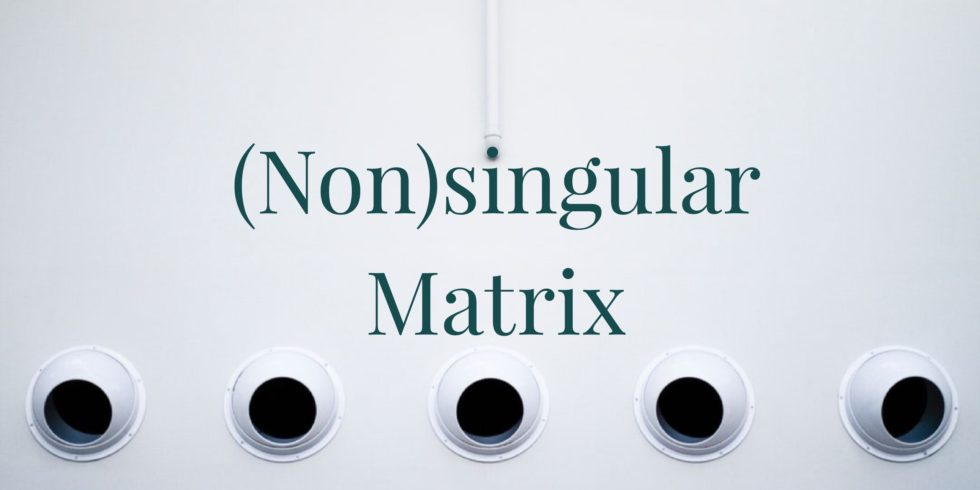# A Condition that a Vector is a Linear Combination of Columns Vectors of a Matrix## Problem 656

Suppose that an $n \times m$ matrix $M$ is composed of the column vectors $\mathbf{b}_1 , \cdots , \mathbf{b}_m$.

Prove that a vector $\mathbf{v} \in \R^n$ can be written as a linear combination of the column vectors if and only if there is a vector $\mathbf{x}$ which solves the equation $M \mathbf{x} = \mathbf{v}$.Add to solve later

## Proof.

Suppose that $\mathbf{v}$ is a linear combination of the vectors $\mathbf{b}_1, \dots, \mathbf{v}_m$. That is, suppose there are coefficients $x_1 , x_2 , \cdots , x_m$ such that
$x_1 \mathbf{b}_1 + x_2 \mathbf{b}_2 + \cdots + x_m \mathbf{b}_m = \mathbf{v}.$

Then define the column vector
$\mathbf{x} = \begin{bmatrix} x_1 \\ x_2 \\ \vdots \\ x_m \end{bmatrix}.$ Then $\mathbf{x}$ satisfies the equation $M \mathbf{x} = \mathbf{v}$.

On the other hand, suppose that there is a vector $\mathbf{x}$ which satisfies the desired equation with components $x_1 , x_2 , \cdots, x_m$.

Then these components can be used to find the linear combination
$x_1 \mathbf{b}_1 + x_2 \mathbf{b}_2 + \cdots + x_m \mathbf{b}_m = M \mathbf{x} = \mathbf{v}.$ Hence, the vector $\mathbf{v}$ is a linear combination of column vectors $\mathbf{b}_1, \dots, \mathbf{b}_m$.Add to solve later

### More from my site

#### You may also like...

This site uses Akismet to reduce spam. Learn how your comment data is processed.

###### More in Linear Algebra##### Show that the Given 2 by 2 Matrix is Singular

Consider the matrix $M = \begin{bmatrix} 1 & 4 \\ 3 & 12 \end{bmatrix}$. (a) Show that $M$ is singular....

Close